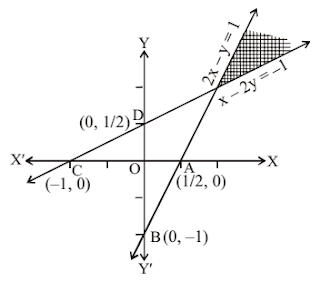Solve the following system of inequalities graphically: 2x – y >1, x – 2y < – 1

Asked by Abhisek | 1 year ago |  96

##### Solution :-

Given 2x – y >1……………… (i)

Putting value of x = 0 and y = 0 in equation one by one, we get value of

y = -1 and x = $$\dfrac{1}{2}$$ = 0.5

The points are (0,-1) and (0.5, 0)

Checking for the origin, putting (0, 0)

0 >1, which is false

Hence the origin does not lie in the solution region. The required region would be on the right of the lines graph.

x – 2y < – 1………… (ii)

Putting value of x = 0 and y = 0 in equation one by one, we get value of

y = $$\dfrac{1}{2}$$ = 0.5 and x = -1

The required points are (0, 0.5) and (-1, 0)

Now checking for the origin, (0, 0)

0 < -1 which is false

Hence the origin does not lies in the solution area, the required area would be on the left side of the lines graph. the shaded area is the required solution of the given inequalities.Answered by Pragya Singh | 1 year ago

### Related Questions

#### Solve each of the following in equations and represent the solution set on the number line

Solve each of the following in equations and represent the solution set on the number line $$\dfrac{5x}{4}-\dfrac{4x-1}{3}>1,$$ where x ϵ R.

#### Solve each of the following in equations and represent the solution set on the number line.

Solve each of the following in equations and represent the solution set on the number line.$$\dfrac{5x-8}{3}\geq \dfrac{4x-7}{2}$$, where x ϵ R.

#### Solve each of the following in equations and represent the solution set on the number line. 3 – 2x ≥ 4x – 9,

Solve each of the following in equations and represent the solution set on the number line. 3 – 2x ≥ 4x – 9, where x ϵ R.

#### Solve each of the following in equations and represent the solution set on the number line. 3x – 4 > x + 6

Solve each of the following in equations and represent the solution set on the number line. 3x – 4 > x + 6, where x ϵ R.